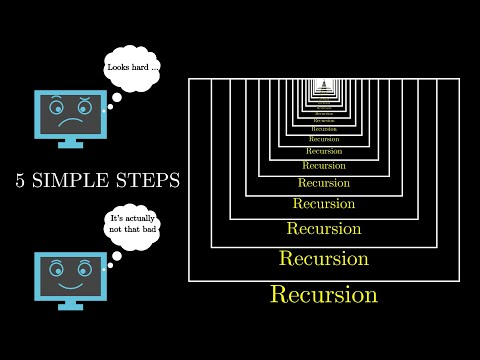Algorithms and Data Structures

• Status
• 1
Implementation
• 2
Bitwise operations
• 3
Prefix Sums
• 4
Sliding window / Two pointers
• 5
Modular Arithmetic
• 6
Number Theory
• 7
Binary Search
• 8
Basic Sorting
• 9
Greedy Algorithms
• 10
Basic Dynamic Programming
• 11
Recursion
• 12
• 13
Queue & Stack
• 14
Binary tree + BST
• 15
Divide & Conquer + Advanced Sorting
• 16
Heap
• 17
Hashing
• 18
Graph Representation
• 19
BFS

• # Recursion

When writing programs we usually call different functions to perform a very specific task. We call `sqrt` to calculate the square root of a number, we call a function `max` or `min` to calculate the maximum or the minimum of numbers. Recursion is the process of calling the same function from itself. So, if we have implemented a function with a name `f` if somewhere in the body of `f`, we call the function `f` again (maybe with different arguments) that would be a recursive call:
``````def f(n):                                   # Define a function named f
print(f'f() called with argument {n}')  # Print on each call (for demonstration)
if n <= 0:                              # Stop if n is negative
print('Let\'s stop here...')
else:                                   # Otherwise call f with a smaller number
f(n - 1)

f(10)``````
This is what the program would print
``````f() called with argument 10
f() called with argument 9
f() called with argument 8
f() called with argument 7
f() called with argument 6
f() called with argument 5
f() called with argument 4
f() called with argument 3
f() called with argument 2
f() called with argument 1
f() called with argument 0
Let's stop here...``````
This is a toy example demonstrating how a very basic recursive function could be implemented. In more real-world scenarios, we usually do some computations inside functions and return values after those computations instead of just printing messages.
In algorithmic problems, recursion can be very useful especially when dealing with graphs, advanced sorting algorithms, or backtracking which we’ll cover later in the course. Recursive problems are very common and sometimes it’s much easier to implement a recursive solution than an iterative one using loops.A video by Reducible - 5 Simple Steps for Solving Any Recursive Problem
As a demonstration of how one might have a computation inside a recursive function, we can try to calculate the sum of all the numbers from `1 … n` given a number `n`:
``````def sum(n):
print(f'sum() called with argument {n}')
if n == 1:         # In case the given number is 1 => the sum is 1 as well
return 1       # Return here and don't execute the following commands
prev = sum(n - 1)  # Compute the sum for 1...(n-1)

print(f'Adding current {n} to prev {prev}')
return n + prev    # Return n + the sum for 1...(n-1)

print(sum(10))``````
This will print all the calls and the number `55` at the end:
``````sum() called with argument 10
sum() called with argument 9
sum() called with argument 8
sum() called with argument 7
sum() called with argument 6
sum() called with argument 5
sum() called with argument 4
sum() called with argument 3
sum() called with argument 2
sum() called with argument 1
Adding current 2 to prev 1
Adding current 3 to prev 3
Adding current 4 to prev 6
Adding current 5 to prev 10
Adding current 6 to prev 15
Adding current 7 to prev 21
Adding current 8 to prev 28
Adding current 9 to prev 36
Adding current 10 to prev 45
55``````
Recursive leap of faith: the great part about recursive solutions is that one can assume that the function works correctly when called with some simple arguments, and then implement the correct behavior for the current arguments. Similar to calling a function like `sqrt`, you can assume that the function works for some smaller/simpler arguments and call those to build the current result on top of those. The only requirement is making sure the function will arrive to the base case (like `n == 1` in our example) otherwise we would have an infinitely running recursive function and might get a StackOverflow!
In the case of calculating the sum of numbers from 1 up to `n`, the recursive leap of faith would be to assume that the function correctly calculates the previous sum (the sum for `n-1` - `prev = sum(n - 1)`) and then implement the rest of the function based on that assumption. So, if the current `n` is 6, we can assume that `sum(5)` would work correctly and therefore return `6` plus the result of `sum(5)` at the end.

### Challenge

Implement a recursive function that would calculate `n!` modulo .

#### Input

The input contains a single integer `n` (1 ≤ n ≤ 1000).

#### Output

The program should print the result of `n!` modulo .

#### Examples

 Input Output 5 120 10 3628800

#### Constraints

Time limit: 1 seconds

Memory limit: 512 MB

Output limit: 1 MB